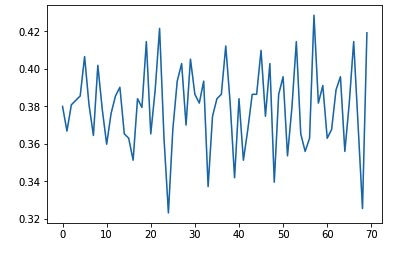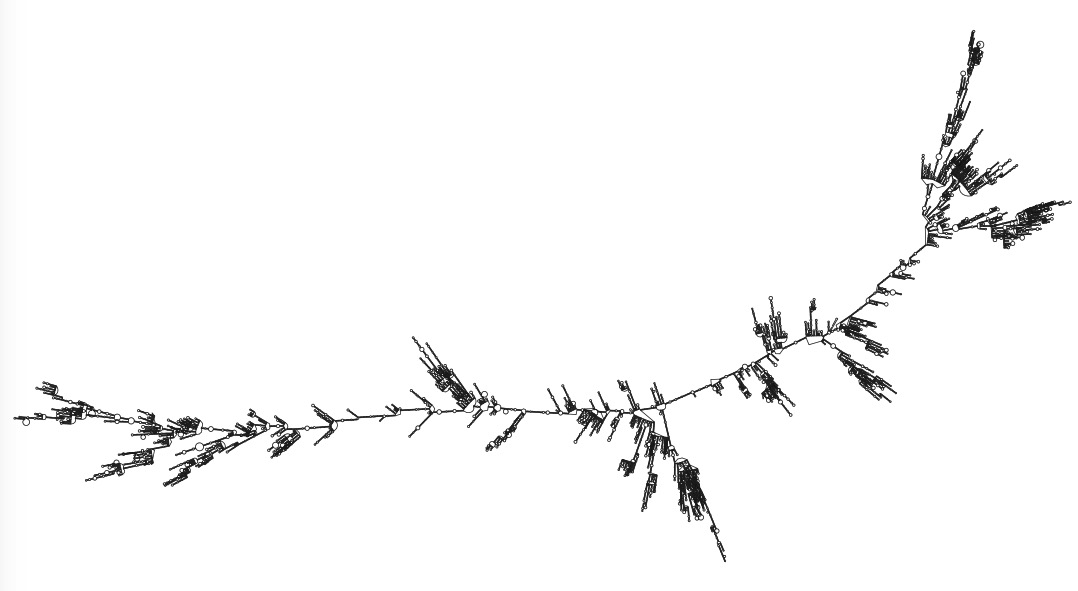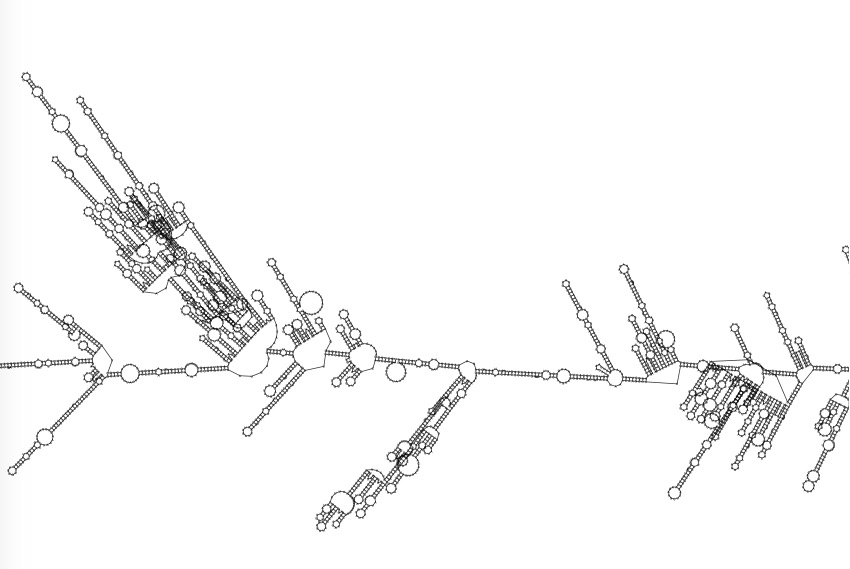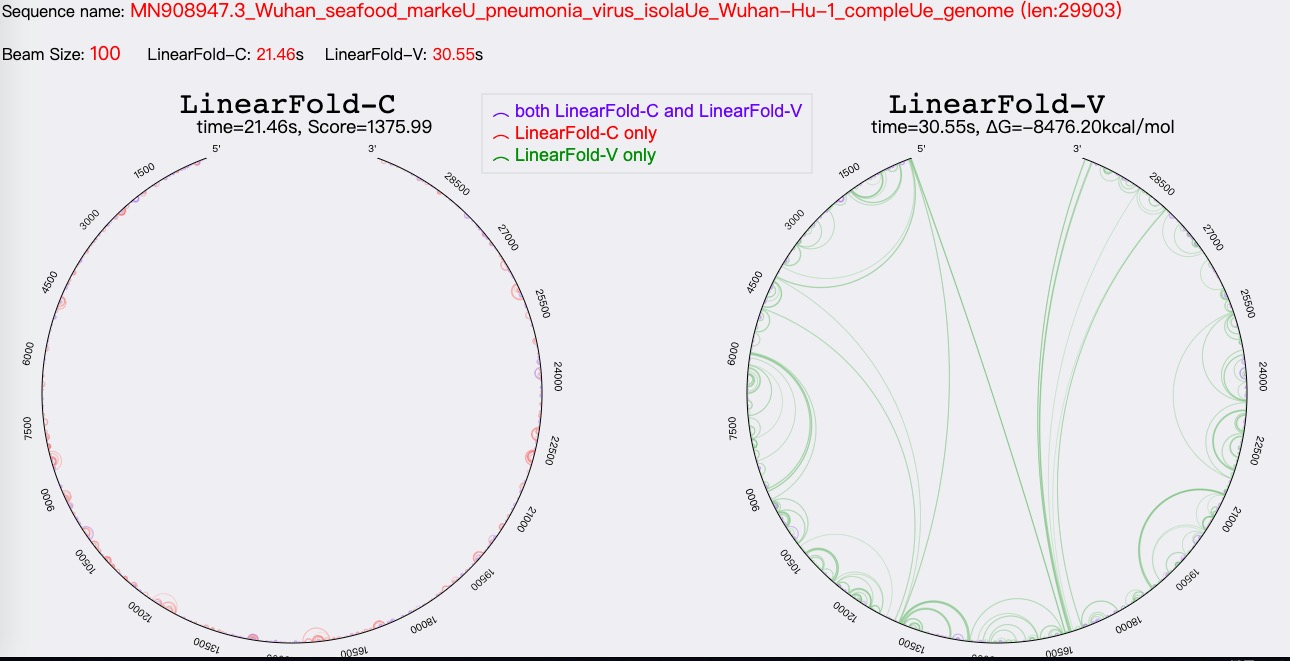# 2019新冠状病毒学习笔记

## >>NEJM官方翻译 | 美国首例新冠病毒肺炎的治疗过程

### 1.序列上的一些探索

``````def readGenome(filename):
#读取全部序列
genome = ''
with open(filename, 'r') as f:
for line in f:
# ignore header line with genome information
if not line == '>':
genome += line.rstrip()
return genome

import collections
collections.Counter(genome)
#Counter({'A': 8954, 'U': 9594, 'G': 5863, 'C': 5492})

import matplotlib.pyplot as plt
def findGCByPos(file):
''' Find the GC ratio at each position in the read '''
# Keep track of the number of G/C bases and the total number of bases at each position
gc =  * 70
totals =  * 71
with open(file) as f:
for line in f:
#print(len(line))
if line.startswith('>'):
continue
for i in range(0,len(line)):
if line[i] == 'C' or line[i] == 'G':
gc[i] += 1
totals[i] += 1
#print(len(line))
# Divide G/C counts by total counts to get the average at each position
for i in range(len(gc)):
if totals[i] > 0:
gc[i] /= float(totals[i])
#print(totals)
return gc

gc = findGCByPos('../Wuhan-RNA.fasta')
plt.plot(range(len(gc)), gc)
plt.show()
``````### 2.RNA折叠

``````#安装这个软件，还是conda
conda install -c bioconda viennarna
RNAfold  < Wuhan-RNA.fasta  > Wuhan-rna.ss
RNAplot > Wuhan-rna.ss
``````### 3.百度linearFold网页服务试用